# Corn and ethanol futures hedge ratios

This entry is part 2 of 2 in the series The Bio-ethanol crush margin

A large amount of literature has been published discussing hedging techniques and a number of different hedging models and statistical refinements to the OLS model that we will use in the following. For a comprehensive review see “Futures hedge ratios: a review,” (Chen et al., 2003).

We are here looking for hedge models and hedge ratio estimations techniques that are “good enough” and that can fit into valuation models using Monte Carlo simulation.

The ultimately purpose is to study hedging strategies using P&L and Balance simulation to forecast the probability distribution for the company’s equity value. By comparing the distributions for the different strategies, we will be able to select the hedging strategy that best fits the boards risk appetite /risk aversion and that at the same time “maximizes” the company value.

Everything should be made as simple as possible, but not simpler. – Einstein, Reader’s Digest. Oct. 1977.

To use futures contracts for hedging we have to understand the objective: a futures contract serves as a price-fixing mechanism. In their simplest form, futures prices are prices set today to be paid in the future for goods. If properly designed and implemented, hedge profits will offset the loss from an adverse price moves. In a like fashion, hedge losses will also eliminate effects of a favorable price change. Ultimately, the success of any hedge program rests on the implementation of a correctly sized futures position.

### The minimum variation hedge

This is often referred to as – the volatility-minimizing hedge for one unit of exposure. It can be found by minimizing the variance of the hedge payoff at maturity.

For an ideal hedge, we would like the change in the futures price (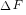) to match as exactly as possible the change in the value of the asset (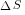) we wish to hedge, i.e.:The expected payoff from the hedge will be equal to the value of the cash position at maturity plus the payoff of the hedge (Johnson, 1960) or: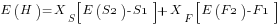With spot position XS, a short futures market holding XF, spot price S1 and expected spot price at maturity E (S2), current future contract price F1 andexpected future price E (F2) – excluding transaction costs.

What we want is to find the value of the futures position that reduces the variability of price changes to the lowest possible level.

The minimum-variance hedge ratio is then defined as the number of futures per unit of the spot asset that will minimize the variance of the hedged portfolio returns.

The variance of the portfolio return is:1:Where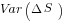is the variance in the future price change,is the variance of the change in the spot price and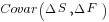the covariance between the spot and future price changes. Letting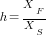represent the proportion of the spot position hedged, minimum value of Var (H) can then be found2 as:Where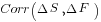is the correlation between the spot and future price changes while  assuming that XS is exogenous determined or fixed.

### Estimating the hedge coefficient

It is also possible to estimate the optimal hedge (h*) using regression analysis. The basic equation is: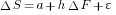withas the change in spot price not explained by the regression model. Since the basic OLS regression for this equation estimates the value of h* as: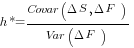we can use this regression to find the solution that minimizes the objective function E(H). This is one of the reasons that use of the objective function E (H) is so appealing.3

We can then use the coefficient of determination, or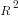, as an estimate of the percentage reduction in the variability of changes in the value of the cash position from holding the hedged position – the hedge effectiveness. (Ederington, 1979)4.

### The basis

Basis is defined as the difference between the spot price (S) and the futures price (F). When the expected change in the future contract price is equal to the expected change in the spot price, the optimal variance minimizing strategy is to set  h*=1. However, for most future contract markets the future price does not perfectly parallel the spot price, causing an element of basis risk to directly affect the hedging decision.

A negative basis is called contango and a positive basis backwardation:

1. When the spot price increases by more than the futures price, the basis increases and is said to “strengthen the basis” (when unexpected, this is favorable for a short hedge and unfavorable for a long hedge).
2. When the futures price increases by more than the spot price, the basis declines and this is said to “weaken the basis” (when unexpected, this is favorable for a long hedge and unfavorable for a short hedge).

There will usually be a different basis for each contract.

### The number of futures contracts

The variance minimizing number of futures contracts N* will be:

N*=h*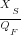Where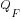is the size of one futures market contract. Since futures contracts are marked to market every day, the daily losses are debited and daily gains credited the parties accounts – settlement variations – i.e. the contracts are closed every day. The account will have to be replenished if the account falls below the maintenance margin (margin call). If the account is above the initial margin withdrawals can be made from the account.

Ignoring the incremental income effects from investing variation margin gains (or borrowing to cover variation margin losses), we want the hedge to generate h*. Appreciating that there is an incremental effect, we want to accrue interest on a “tailed” hedge such that (Kawaller, 1997):

h*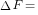or
ĥ = h*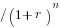or h*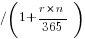if time to maturity is less than one year.

Where:
r = interest rate and
n = number of days remaining to maturity of the futures contract.

This amounts to adjusting the hedge by a present value factor. Tailing converts the futures position into a forward position. It negates the effect of daily resettlement, in which profits and losses are realized before the day the hedge is lifted.

For constant interest rates the tailed hedge (for h* < 1.) rises over time to reach the exposure at the maturity of the hedge. Un-tailed the hedge will over-hedge the exposure and increase the hedger’s risk.  Tailing the hedge is especially of importance when the interest rate is high and the time to maturity long.

An appropriate interest rate would be one that reflects the average of the firm’s cost of capital (WACC) and the rate it would earn on its investments (ROIC) both which will be stochastic variable in the simulation. The first would be relevant in cases when the futures contracts generate losses, while the second when the futures contracts generate gains. In practice some average of these rates are used.5
There are traditionally two approaches to tailing:

1. Re-balance the tail each day. In this case the tailed hedge ratio is adjusted each day to maturity of the futures contract. In this approach the adjustment declines each day, until at expiration there is no adjustment.
2. Use a constant tail (average): ĥ= h*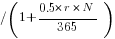where N is the original number of days remaining to maturity. In this shortcut, the adjustment is made at the time the hedge is put on, and not changed. The hedge will start with being too big and ends with being too small, but will on average be correct.

For investors where trading is active, the first approach is more convenient, for inactive traders, the second is often used.

Since our models always incorporate stochastic interest rates, hedges discounted with the appropriate rates are calculated. This amounts to solving the set of stochastic simultaneous equations created by the hedge and the WACC/ROIC calculations since the hedges will change their probability distributions. Note that the tailed hedge ratio will be a stochastic variable, and that minimizing the variance of the hedge will not necessarily maximize the company value. The value of – ĥ – that maximizes company value can only be found by simulation given the board’s risk appetite / risk aversion.

### The Spot and Futures Price movements

At any time there are a number of futures contracts for the same commodity simultaneously being priced. The only difference between them is the delivery month. A continuous contract takes the individual contracts in the futures market and splices them together. The resulting continuous series6 allows us to study the price history in the market from a single chart. The following graphs show the price movements7 for the spliced corn contracts C-2010U to 2011N and the spliced ethanol contracts EH-2010U to 2011Q.

In the graphs the spot price is given by the blue line and the corresponding futures price by the red line.

For the corn futures, we can see that there is a difference between the spot and the futures price – the basis8  – but that the price movements of the futures follow the spot price closely or – vice versa.The spliced contracts for bioethanol are a little different from the corn contracts. The delivery location is the same and the curves are juxtaposed very close to each other. Here are however other differences.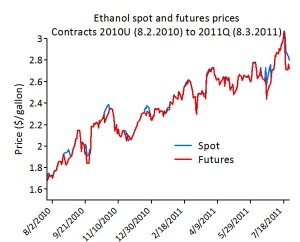## The regression – the futures assay

The selected futures contracts give us five parallel samples for the relation between the corn spot and futures price, and six for the relation between the ethanol spot and ethanol futures price. For every day in the period 8/2/2010 to 7/14/2011 we have from one to five observations of the corn relation (five replications) and from 8/5/2010 to 8/3/2011 we have one to twelve observations of the ethanol relation. Since we follow a set of contracts, the number of daily observations of the corn futures prices starts with five (twelve for the ethanol futures) and ends with only one as the contracts matures.  We could of course also have selected a sample giving an equal number of observations every day.

There are three likely models which could be fit:

1. Simple regression on the individual data points,
2. Simple regression on the daily means,and
3. Weighted regression on the daily means using the number of observations as the weight.

When the number of daily observations is equal all three models will have the same parameter estimates. The weighted and individual regressions will always have the same parameter estimates, but when the sample sizes are unequal these will be different from the unweighted means regression. Whether the weighted or unweighted model should be used when the number of daily observations is unequal will depend on the situation.

Since we now have replications of the relation between spot and the futures price we have the opportunity to test for lack of fit from the straight line model.

In our case using this approach have a small drawback. We are looking for the regression of the spot price changes against the price changes in the futures contract. This model however will give us the inverse: the regression of the price changes in the futures contract against the changes in spot price. The inverse of the slope of this regression, which is what we are looking for, will in general not give the correct answer (Thonnard, 2006).  So we will use this approach (model#3) to test for linearity and then model #1 with all data for estimation of the slope.

Ideally we would like to find stable (efficient) hedge ratios in the sense that they can be used for more than one hedge and over a longer period of time, thus greatly simplifying the workload for ethanol producing companies.

All prices, both spot and futures in the following, have been converted from \$/gallon (ethanol) or \$/bushel (corn) to \$/kg.

### The Corn hedge ratio

The analysis of variance table (ANOVA) for the weighted regression of the changes in the corn futures prices on the changes in corn spot prices (model#3):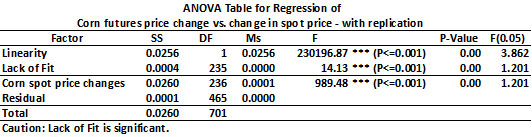The analysis of variance cautions us that the lack of fit to a linear model for all contracts is significant. However the sum of squares due to this is very small compared to the sum of squares due to linearity – so we will regard the changes in the futures prices to have been generated by a linear function of the changes in the spot prices and the hedge ratios found as efficient. In figure below the circles gives the daily means of the contracts and the line the weighted regression on these means: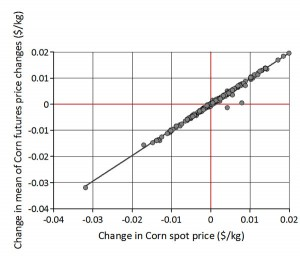Nevertheless, this linear model will have to be monitored closely as further data becomes available.

The result from the parameter estimation using simple regression (model#1) is given in the table below: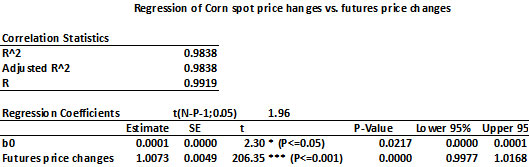The relation is: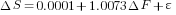Giving the un-tailed corn hedge ratio h* = 1.0073

First, since the adjusted  R-square value (0.9838) is an estimate of the percentage reduction in the variability of changes in the value of the cash position from holding the hedged position, a hedge based on this regression coefficient (slope) should be highly effective.

The ratio of the variance of the hedged position and the un-hedged position is equal to 1-R2.  The variance of a hedged position based on this hedge ratio will be 12.7 % of the unhedged position.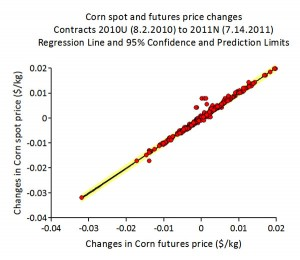We have thus eliminated 87.3 % of the variance of the unhedged position. For a simple model like this this can be considered as a good result.

In the figure the thick black line gives the 95% confidence limits and the yellow area the 95% prediction limits. As we can see, the relationship between the daily price changes is quite tight thus promising the possibility of effective hedges.

Second, due to the differencing the basis caused by the difference in delivery location have disappeared, and even if the constant term is significant, it is so small that it with little loss can be considered zero.

The R-square values would have been higher for the regressions on the means than for the regression above. This is because the total variability in the data would have been reduced by using means (note that the total degrees of freedom is reduced for the regressions on means).  A regression on the means will thus always suggest greater predictive ability than a regression on individual data because it predicts mean values, not individual values.

### The Ethanol hedge ratio

The analysis of variance table (ANOVA) for the weighted regression of the changes in the ethanol futures prices on the changes in ethanol spot prices (model#3):The analysis of variance again cautions us that the lack of fit to a linear model for all contracts is significant.  In this case it is approximately ten times higher than for the corn contracts.

However the sum of squares due to this is small small compared to the sum of squares due to linearity – so we will regard the changes in the futures prices to have been generated by a close to linear function of the changes in the spot prices and the hedge ratios found as “good enough”. In figure below the circles gives the daily means of the contracts and the line the weighted regression on these means: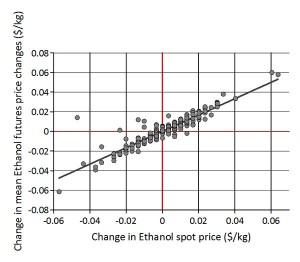In this graph we can clearly see the deviation from a strictly linear model. The assumption of a linear model for the changes in ethanol spot and futures prices will have to be monitored very closely as further data becomes available.

The result from the parameter estimation using simple regression (model#1) is given in the table below: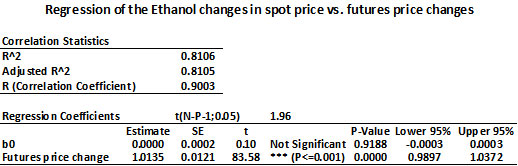The relation is: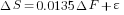Giving the un-tailed corn hedge ratio h* = 1.0135

The adjusted  R-square value (0.8105) estimating the percentage reduction in the variability of changes in the value of the cash position from holding the hedged position is high even with the “lack of linearity”. A hedge based on this regression coefficient (slope) should then still be highly effective.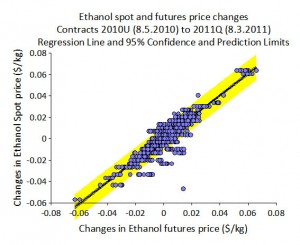The variance of a hedged position based on this hedge ratio will be 43.7 % of the unhedged position. It is not as good as for the corn contracts, but will still give a healthy reduction in the ethanol price risk facing the company.

As this turned out, we can use both of these estimation methods for the hedge ratio as basis for strategy simulations, but one question remains unanswered: will this minimize the variance of the crush  ratio?

### References

Understanding Basis, Chicago Board of Trade, 2004.  http://www.gofutures.com/pdfs/Understanding-Basis.pdf

Bond, Gary E. (1984). “The Effects of Supply and Interest Rate Shocks in Commodity Futures Markets,” American Journal of Agricultural Economics, 66, pp. 294-301.

Chen, S. Lee, C.F. and Shrestha, K (2003) “Futures hedge ratios: a review,” The Quarterly Review of Economics and Finance, 43 pp. 433–465

Ederington, Louis H. (1979). “The Hedging Performance of the New Futures Markets,” Journal of Finance, 34, pp. 157-70

Einstein, Albert (1923). Sidelights on Relativity (Geometry and Experience). P. Dutton., Co.

Figlewski, S., Lanskroner, Y. and Silber, W. L. (1991) “Tailing the Hedge: Why and How,” Journal of Futures Markets, 11: pp. 201-212.

Johnson, Leland L.  (1960). ” The Theory of Hedging and Speculation in Commodity Futures,” Review of Economic Studies, 27, pp. 139-51.

Kawaller, I. G. (1997 ) ”Tailing Futures Hedges/Tailing Spreads,” The Journal of Derivatives, Vol. 5, No. 2, pp. 62-70.

Li, A. and Lien, D. D. (2003) “Futures Hedging Under Mark-to-Market Risk,” Journal of Futures Markets, Vol. 23, No. 4.

Myers Robert J. and Thompson Stanley R. (1989) “Generalized Optimal Hedge Ratio Estimation,” American Journal of Agricultural Economics, Vol. 71, No. 4, pp. 858-868.

Thonnard, M., (2006), Confidence Intervals in Inverse Regression. Diss. Technische Universiteit Eindhoven, Department of Mathematics and Computer Science, Web. 5 Apr. 2013. <http://alexandria.tue.nl/extra1/afstversl/wsk-i/thonnard2006.pdf>.

Stein, Jerome L.  (1961). “The Simultaneous Determination of Spot and Futures Prices,” American Economic Review, 51, pp. 1012-25.

### Endnotes

Series Navigation<< The probability distribution of the bioethanol crush margin
1. The variance of the un-hedged position is: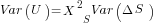[]
2. by minimizing Var (H) as a function of h []
3. Note that other and very different objective functions could have chosen. []
4. Not taking into account variation margins etc. []
5. See FAS 133 and later amendments []
6. The simplest method of splicing is to tack successive delivery months onto each other. Although the prices in the history are real, the chart will also preserve the price gaps that are present between expiring deliveries and those that replace them. []
7. To avoid price gap problems, many prefer to base analysis on adjusted contracts that eliminate roll-over gaps. There are two basic ways to adjust a series.
Forward-adjusting works by beginning with the true price for the first delivery and then adjusting each successive set of prices up or down depending on whether the roll-over gap is positive or negative.
Back-adjusting reverses the process. Current price are always real but historical prices are adjusted up or down. This is the often preferred method, since the series always will show the latest actual price. However, there is no perfect method producing a continuous price series satisfying all needs. []
8. The reasons for the price difference are transportation costs between delivery locations, storage costs and availability, and variations between local and worldwide supply and demand of a given commodity. In any event, this difference in price plays an important part in what is being actually pay for the commodity when you hedge. []